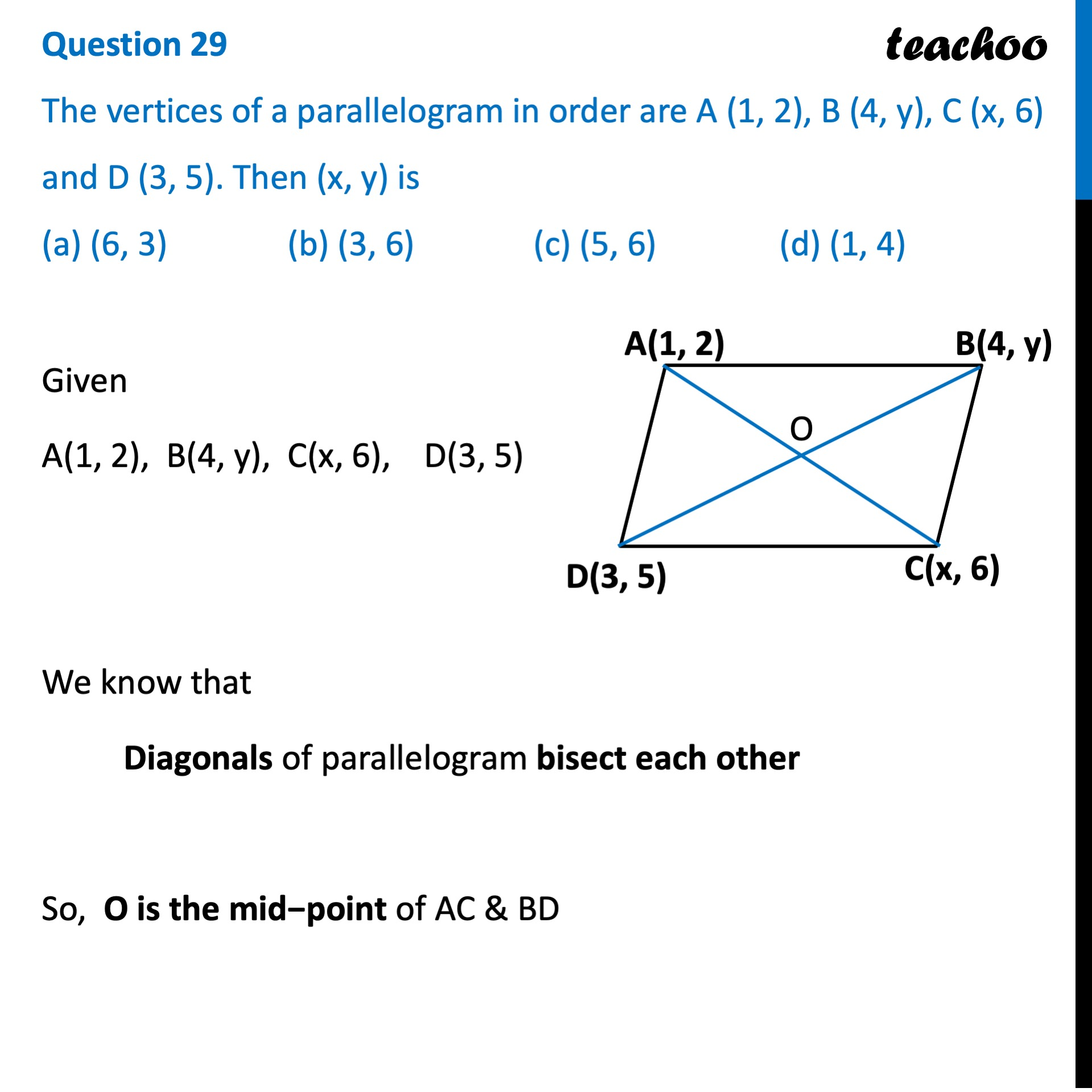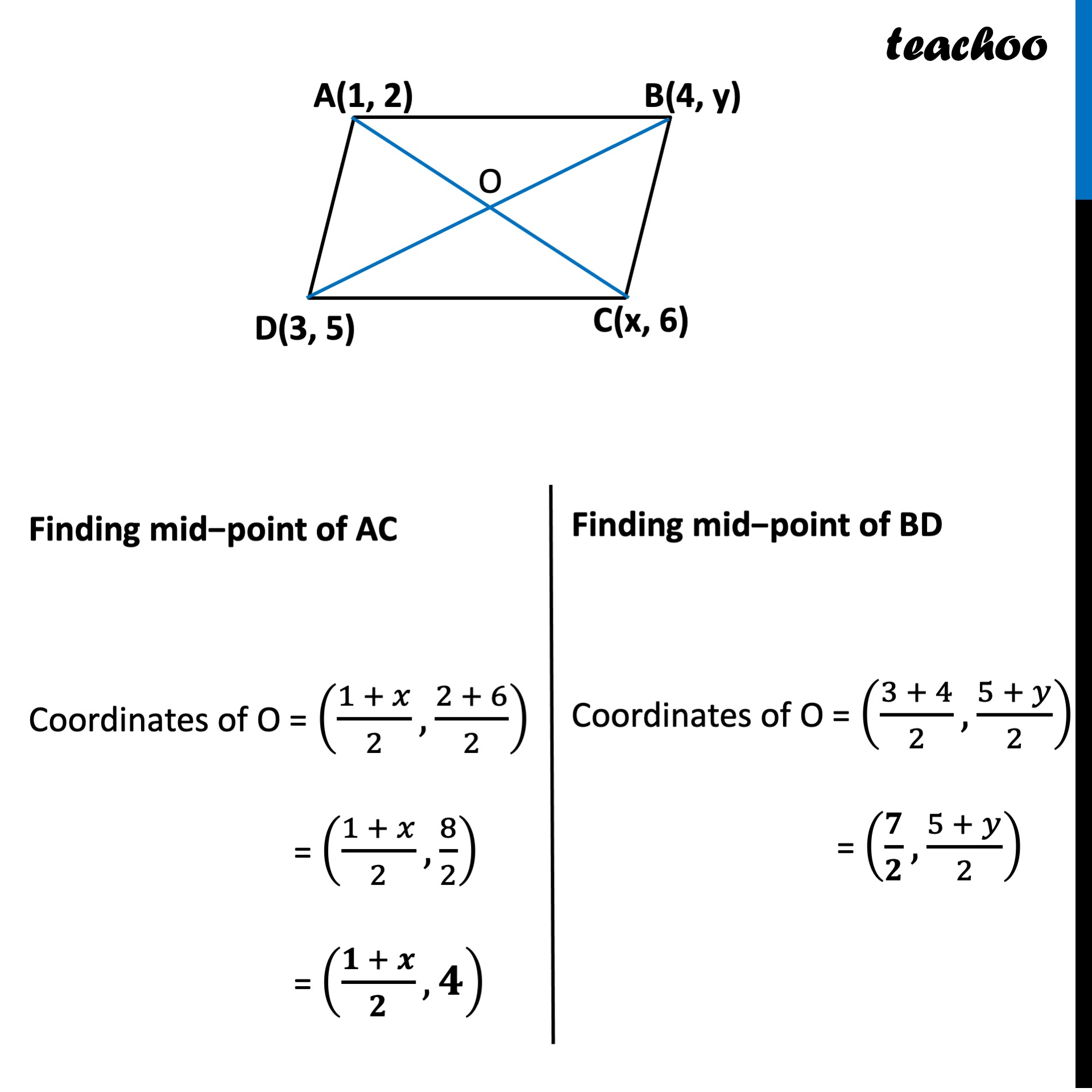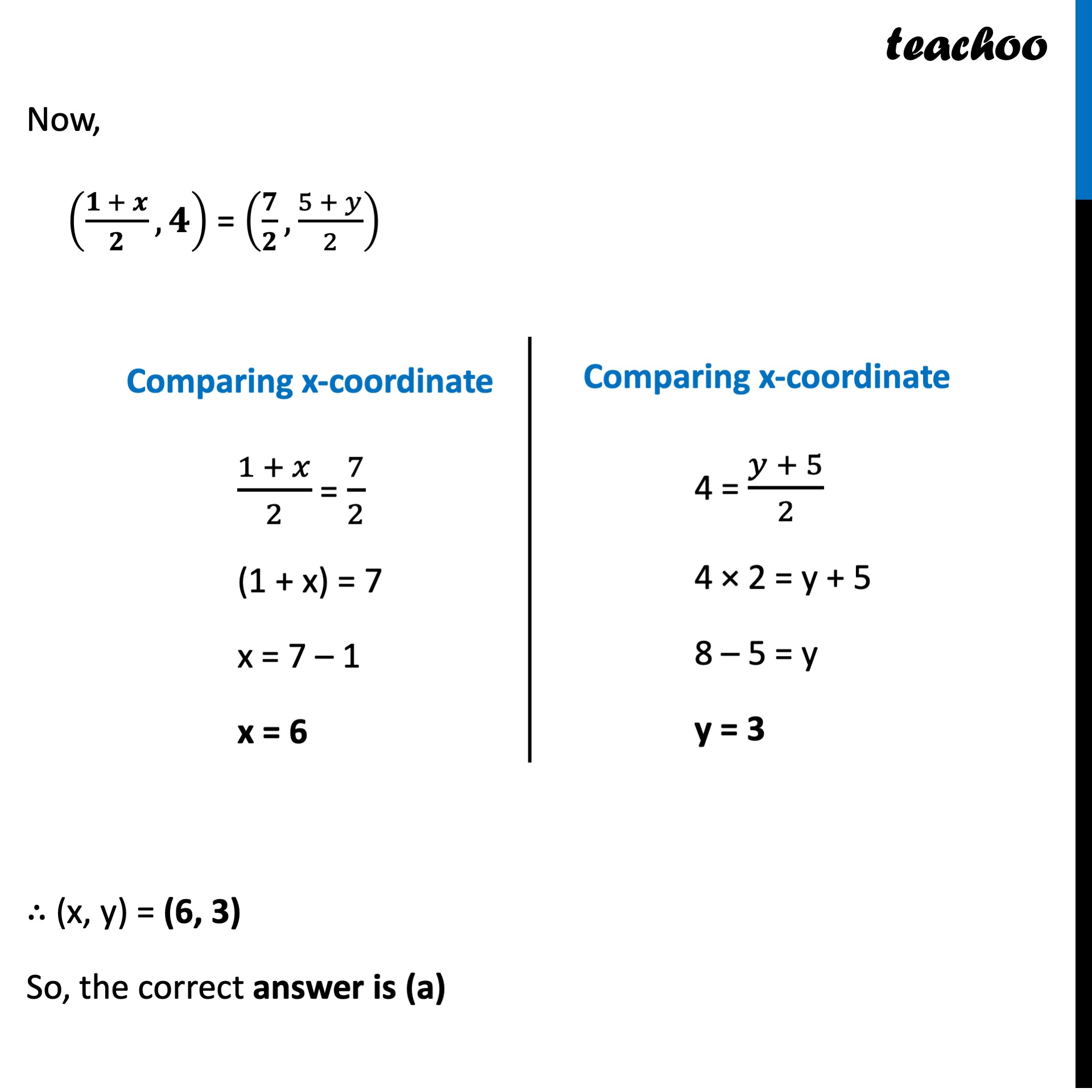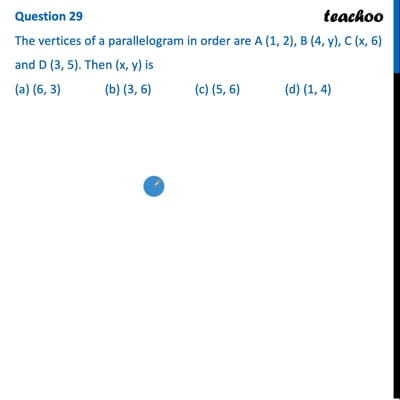CBSE Class 10 Sample Paper for 2022 Boards - Maths Standard [MCQ]

Class 10
Solutions of Sample Papers for Class 10 Boards

## (a) (6, 3)   (b) (3, 6)   (c) (5, 6)   (d) (1, 4)

This question is inspired from Ex 7.2, 6 (NCERT) - Chapter 7 Class 10 - Coordinate GeometryThis video is only available for Teachoo black users

Get live Maths 1-on-1 Classs - Class 6 to 12

### Transcript

Question 29 The vertices of a parallelogram in order are A (1, 2), B (4, y), C (x, 6) and D (3, 5). Then (x, y) is (a) (6, 3) (b) (3, 6) (c) (5, 6) (d) (1, 4) Given A(1, 2), B(4, y), C(x, 6), D(3, 5) We know that Diagonals of parallelogram bisect each other So, O is the mid−point of AC & BD Finding mid−point of AC Coordinates of O = ((1 + 𝑥)/2,(2 + 6)/2) = ((1 + 𝑥)/2,8/2) = ((𝟏 + 𝒙)/𝟐,𝟒) Finding mid−point of BD Coordinates of O = ((3 + 4)/2,(5 + 𝑦)/2) = (𝟕/𝟐,(5 + 𝑦)/2) Now, ((𝟏 + 𝒙)/𝟐,𝟒) = (𝟕/𝟐,(5 + 𝑦)/2) Comparing x-coordinate (1 + 𝑥)/2 = 7/2 (1 + x) = 7 x = 7 – 1 x = 6 Comparing x-coordinate 4 = (𝑦 + 5)/2 4 × 2 = y + 5 8 – 5 = y y = 3 ∴ (x, y) = (6, 3) So, the correct answer is (a)